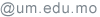Che Man CHENG, Raymond鄭智文 Associate Professor Associate Head of Department of Mathematics (MAT)

Academic Qualifications | Working Experience | Teaching | Research | Selected Publications | Contact Details

### Academic Qualification

• PhD (Mathematics), The University of Hong Kong, Hong Kong (1991)
• B.Sc. (Mathematics), The University of Hong Kong, Hong Kong (1987)

### Working Experience

 2016- Associate Head of Department of Mathematics, FST, UM 2000- Associate professor, FST, UM 2002-2005, Head of Department of Mathematics, FST, UM 1999-2001, Member of the Management Committee of Faculty of Education, UM 1997-2002, Coordinator of Mathematics program, FST, UM 1992-2000, Assistant professor, FST, UM

### Teaching

#### B.Sc. Courses

1. Abstract Algebra I (EDUC350)
2. Abstract Algebra II (EDUC351)
3. Calculus I (MATB110)
4. Calculus II (MATB120)
5. Complex Analysis (EDUC354)
6. Linear Algebra (MATH103)
7. Operations Research (MATB226)
8. Operations Research I (SFTW122)
9. Operational Research (EDUC413)
10. Quantitative Reasoning for Science and Technology (GEST1004)
11. Real Analysis I (EDUC204)
12. Mathematical Analysis I (MATH101)
13. Mathematical Analysis II (MATH102)
14. Mathematical Analysis III (MATB350)

#### M.Sc. Courses

1. Algebra (IMMT110)
2. Matrix Analysis (IMMT125)
3. Matrix Analysis (IMAT008)

### Research

#### Research Interests

• Matrix theory, including numerical ranges, eigenvalues and singular values, norms of commutators, and Hurwitz matrix equations

### Selected Publications

1. C.-M. Cheng, A note on interlacing polynomial, Southeast Asian Bull. Math14 (1990), 25-26.
2. C.-M. Cheng, Two results on C-congruence numerical radii, Tamkang J. Math21 (1990), 59-64.
3. C.-M. Cheng, An approximation problem with respect to symmetric gauge functions with applications to matrix spaces, Linear and Multilinear Algebra29 (1991), 169-180.
4. Y.-H. Au-Yeung, C. M. Cheng, Permutation matrices whose convex combinations are orthostochastic, Linear Algebra Appl150 (1991), 243-253.
5. C.-M. Cheng, Independence of eigenvalues and independence of singular values of submatrices, Linear Algebra Appl166 (1992), 115-129.
6. C.-M. Cheng, Cases of equality for a singular value inequality of the Hadamard product, Linear Algebra Appl177 (1992), 209-231.
7. Y.-H. Au-Yeung, C.-M. Cheng, Two formulas for the Radon-Hurwitz number, Linear and Multilinear Algebra34 (1993), 59-65.
8. C.-M. Cheng, On the decomposable numerical range of λI-N, Linear and Multilinear Algebra37 (1994), 197-205.
9. C.-M. Cheng, C.-K. Li, Some geometrical properties of the decomposable numerical range, Linear and Multilinear Algebra37 (1994), 207-212.
10. C.-M. Cheng, On principal sections of a pair of forms, Proc. Amer. Math. Soc123 (1995), 2949-2954.
11. C.-M. Cheng, C. K. Li, On the Hu-Hurley-Tam conjecture concerning the generalized numerical range, Linear Algebra Appl305 (2000), 87-97.
12. C.-M. Cheng, R. A. Horn, C.-K. Li, Inequalities and equalities for the Cartesian decomposition of complex matrices, Linear Algebra Appl. 341 (2002), 219-237.
13. C.-M. Cheng, X-Q Jin, Some stability properties of T. Chan’s preconditioner, Linear Algebra Appl.395 (2005), 361-365.
14. C.-M. Cheng, X.-Q. Jin, Y-M Wei, Stability properties of superoptimal preconditioner from numerical range, Numer. Linear Algebra Appl. 13 (2006), 513-521.
15. Y.-H. Au-Yeung, C.-M. Cheng, On the pure imaginary quaternionic solutions of the Hurwitz matrix equations, Linear Algebra Appl. 419 (2006), 630-642.
16. C.-M. Cheng, X.-Q. Jin, V.-K. Sin, Stability properties of T. Chan’s preconditioner from numerical range, Numerical Mathematics: A Journal of Chinese Universities (English series) 16 (2007), 28-36.
17. C. M. Cheng, X.-Q. Jin, S.-W. Vong, W. Wang, A note on spectra of optimal and superoptimal preconditioned matrices, Linear Algebra Appl. 422 (2007), 482-485.
18. C.-M. Cheng, I.-C. Law, S.-I Leong, Eigenvalue equalities for ordinary and Hadamard products of powers of positive semidefinite matrices, Linear Algebra Appl. 422 (2007), 771-787.
19. C.-M. Cheng, K.-L. Cheok, I.-T. Leong, 3×3 pure imaginary quaternionic solutions of the Hurwitz matrix equations, Linear and Multilinear Algebra 58 (2010), 863-874.
20. C.-M. Cheng, S.-W. Vong, D. Wenzel, Commutators with maximal Frobenius norm, Linear Algebra Appl432 (2010), 292-306.
21. K.-S. Fong, C.-M. Cheng, I.-K. Lok, Another unitarily invariant norm attaining the minimum norm bound for commutators, Linear Algebra Appl433 (2010), 1793-1797.
22. C.-M. Cheng, K.-S. Fong, I.-K. Lok, Another proof for commutators with maximal Frobenius norm, Recent Advances in Scientific Computing and Matrix Analysis, p. 9-14, Editors: X.-Q. Jin, H.-W. Sun, S.-W. Vong, International Press, 2011.
23. K.-S. Fong, I.-K. Lok, C.-M. Cheng, A note on the norm of commutator and the norm of XY-YX^T, Linear Algebra Appl435 (2011), 1191-1201.
24. C.-M. Cheng, K.-S. Fong, W.-F. Lei, On some norm inequalities involving the commutator and XY-YX^T, Linear Algebra Appl438 (2013), 2793-2807.
25. C.-M. Cheng, Y. Gao, A note on numerical range and product of matrices, Linear Algebra Appl438 (2013), 3139-3143. Corrigendum, Linear Algebra Appl459 (2014), 622-624.
26. C.-M. Cheng, X.-Q. Jin, Matrix decomposition, Encyclopedia of Social Network Analysis and Mining, p. 880-890, Springer Science and Business Media, New York, 2014. ISBN 978-1-4614-6169-2
27. R. Alizadeh, M. B. Asadi, C.-M. Cheng, W. Hong, C.-K. Li, Spectral radius, numerical radius, and the product of operators, J. Math. Anal. Appl423 (2015), 639-645.
28. C.-M. Cheng, X.-Q. Jin, S.-W. Vong, A survey on the Böttcher-Wenzel conjecture and related problems, Oper. Matrices9 (2015), 659-673.
29. C.-M. Cheng, C. Lei, On Schatten p-norms of commutators, Linear Algebra Appl484 (2015), 409-434.
30. C.-M. Cheng, Y. Liang, Some sharp bounds for the commutator of real matrices, Linear Algebra Appl521 (2017), 263-282.
31. Z.-J. Xie, C.-M. Cheng, X. Jin, Some norm inequalities for commutators of contracted tensor products, Linear Algebra Appl. 540 (2018), 244-256.
32. C.-M. Cheng, Y. Liang, Singular values, eigenvalues and diagonal elements of the commutator of 2×2 rank one matrices, Electron. J. Linear Algebra36 (2020), 1-20.
33. C.-M. Cheng, R. Jiao, Proof of Wenzel’s conjecture concerning singular values of the commutator of rank one matrices, Linear Algebra Appl592 (2020), 165-174.

### Contact Details

Faculty of Science and Technology
University of Macau, E11
Avenida da Universidade, Taipa,
Macau, China

Room: E11-3065
Telephone: (853) 8822-4457
Fax: (853) 8822-2426
Email: fstcmc